MathematicsSolving ODE Problems

This section uses the van der Pol equation

•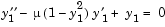to describe the process for solving initial value ODE problems using the ODE solvers.

Example: Solving an IVP ODE (van der Pol Equation, Nonstiff)

This example explains and illustrates the steps you need to solve an initial value ODE problem.

1. Rewrite the problem as a system of first-order ODEs. Rewrite the van der Pol equation (second-order)
•1. where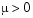is a scalar parameter, by making the substitution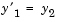. The resulting system of first-order ODEs is

•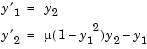1. Code the system of first-order ODEs. Once you represent the equation as a system of first-order ODEs, you can code it as a function that an ODE solver can use. The function must be of the form
• dydt = odefun(t,y)

1. Although t and y must be the function's first two arguments, the function does not need to use them. The output dydt, a column vector, is the derivative of y.

The code below represents the van der Pol system in the function, vdp1. The vdp1 function assumes that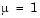.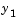and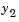become elements y(1) and y(2) of a two-element vector.

• function dydt = vdp1(t,y)
dydt = [y(2); (1-y(1)^2)*y(2)-y(1)];


Note that, although vdp1 must accept the arguments t and y, it does not use t in its computations.

1. Apply a solver to the problem. Decide which solver you want to use to solve the problem. Then call the solver and pass it the function you created to describe the first-order system of ODEs, the time interval on which you want to solve the problem, and an initial condition vector. See Initial Value Problem Solvers and the ODE solver reference page for descriptions of the ODE solvers.
1. For the van der Pol system, you can use ode45 on time interval [0 20] with initial values y(1) = 2 and y(2) = 0.

• [t,y] = ode45(@vdp1,[0 20],[2; 0]);


This example uses @ to pass vdp1 as a function handle to ode45. The resulting output is a column vector of time points t and a solution array y. Each row in y corresponds to a time returned in the corresponding row of t. The first column of y corresponds to, and the second column to.

 Note    See the function_handle (@), func2str, and str2func reference pages, and the Function Handles chapter of "Programming and Data Types" in the MATLAB documentation for information about function handles.

1. View the solver output. You can simply use the plot command to view the solver output.
• plot(t,y(:,1),'-',t,y(:,2),'--')
title('Solution of van der Pol Equation, \mu = 1');
xlabel('time t');
ylabel('solution y');
legend('y_1','y_2')1. As an alternative, you can use a solver output function to process the output. The solver calls the function specified in the integration property OutputFcn after each successful time step. Use odeset to set OutputFcn to the desired function. See OutputFcn for more information.

Passing Additional Parameters to an ODE Function

The solver passes any input parameters that follow the options argument to the ODE function and any function you specify in options. For example:

1. Generalize the van der Pol function by passing it a mu parameter, instead of specifying a value for mu explicitly in the code.
• function dydt = vdp1(t,y,mu)
dydt = [y(2); mu*(1-y(1)^2)*y(2)-y(1)];

2. Pass the parameter mu to the function vdp1 by specifying it after the options argument in the call to the solver. This example uses options = [] as a placeholder.
• [t,y] = ode45(@vdp1,tspan,y0,[],mu)

1. calls

• vdp1(t,y,mu)


See the vdpode code for a complete example based on these functions.

Example: The van der Pol Equation, µ = 1000 (Stiff)

This example presents a stiff problem. For a stiff problem, solutions can change on a time scale that is very short compared to the interval of integration, but the solution of interest changes on a much longer time scale. Methods not designed for stiff problems are ineffective on intervals where the solution changes slowly because they use time steps small enough to resolve the fastest possible change.

When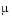is increased to 1000, the solution to the van der Pol equation changes dramatically and exhibits oscillation on a much longer time scale. Approximating the solution of the initial value problem becomes a more difficult task. Because this particular problem is stiff, a solver intended for nonstiff problems, such as ode45, is too inefficient to be practical. A solver such as ode15s is intended for such stiff problems.

The vdp1000 function evaluates the van der Pol system from the previous example, but with= 1000.

• function dydt = vdp1000(t,y)
dydt = [y(2); 1000*(1-y(1)^2)*y(2)-y(1)];


 Note    This example hardcodesin the ODE function. The vdpode example solves the same problem, but passes a user-specifiedas an additional argument to the ODE function. See Additional ODE Solver Arguments.

Now use the ode15s function to solve the problem with the initial condition vector of [2; 0], but a time interval of [0 3000]. For scaling purposes, plot just the first component of y(t).

• [t,y] = ode15s(@vdp1000,[0 3000],[2; 0]);
plot(t,y(:,1),'-');
title('Solution of van der Pol Equation, \mu = 1000');
xlabel('time t');
ylabel('solution y_1');Note    For detailed instructions for solving an initial value ODE problem, see Example: Solving an IVP ODE (van der Pol Equation, Nonstiff).

Evaluating the Solution at Specific Points

The numerical methods implemented in the ODE solvers produce a continuous solution over the interval of integration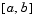. You can evaluate the approximate solution,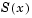, at any point inusing the function deval and the structure sol returned by the solver.

• Sxint = deval(sol,xint)


The ODE solvers return the structure sol when called with a single output argument.

The deval function is vectorized. For a vector xint, the ith column of Sxint approximates the solution.Initial Value Problem Solvers Changing ODE Integration Properties